Documentation

Signal Analyzer

Visualize and compare multiple signals and spectra

Description

The Signal Analyzer app is an interactive tool for visualizing, preprocessing, measuring, analyzing, and comparing signals in the time domain, in the frequency domain, and in the time-frequency domain. Using the app, you can:

• Easily access all the signals in the MATLAB® workspace

• Smooth, filter, resample, detrend, duplicate, extract, and rename signals without leaving the app

• Add and apply custom preprocessing functions

• Visualize and compare multiple waveform, spectrum, persistence, spectrogram, and scalogram representations of signals simultaneously

• Label signals interactively for analysis or for machine learning and deep learning applications

The Signal Analyzer app provides a way to work with many signals of varying durations at the same time and in the same view.

For more information, see Using Signal Analyzer App.

You need a Wavelet Toolbox™ license to use the scalogram view.Open the Signal Analyzer App

• MATLAB Toolstrip: On the Apps tab, under Signal Processing and Communications, click the app icon.

• MATLAB command prompt: Enter signalAnalyzer.

Examples

expand all

Implement a basic digital music synthesizer and use it to play a traditional song in a three-voice arrangement. Specify a sample rate of 2 kHz. Save the song as a MATLAB® timetable.

fs = 2e3;
t = 0:1/fs:0.3-1/fs;

l = [0 130.81 146.83 164.81 174.61 196.00 220 246.94];
m = [0 261.63 293.66 329.63 349.23 392.00 440 493.88];
h = [0 523.25 587.33 659.25 698.46 783.99 880 987.77];
note = @(f,g) [1 1 1]*sin(2*pi*[l(g) m(g) h(f)]'.*t);

mel = [3 2 1 2 3 3 3 0 2 2 2 0 3 5 5 0 3 2 1 2 3 3 3 3 2 2 3 2 1]+1;
acc = [3 0 5 0 3 0 3 3 2 0 2 2 3 0 5 5 3 0 5 0 3 3 3 0 2 2 3 0 1]+1;

song = [];
for kj = 1:length(mel)
song = [song note(mel(kj),acc(kj)) zeros(1,0.01*fs)];
end
song = song'/(max(abs(song))+0.1);

% To hear, type sound(song,fs)

tune = timetable(seconds((0:length(song)-1)'/fs),song);

Open Signal Analyzer and drag the timetable from the Workspace browser to the Signal table. Click Display Grid ▼ to create a two-by-two grid of displays. Select the top two displays and the lower left display and click the Spectrum button to add a spectrum view. Select the lower right display, click Time-Frequency to add a spectrogram view, and click Time to remove the time view. Drag the song to all four displays. Select the lower right display, and in the Spectrogram tab, specify a time resolution of 0.31 second (310 ms) and 0% overlap between adjoining segments. Set the Power Limits to $-50$ dB and $-10$ dB.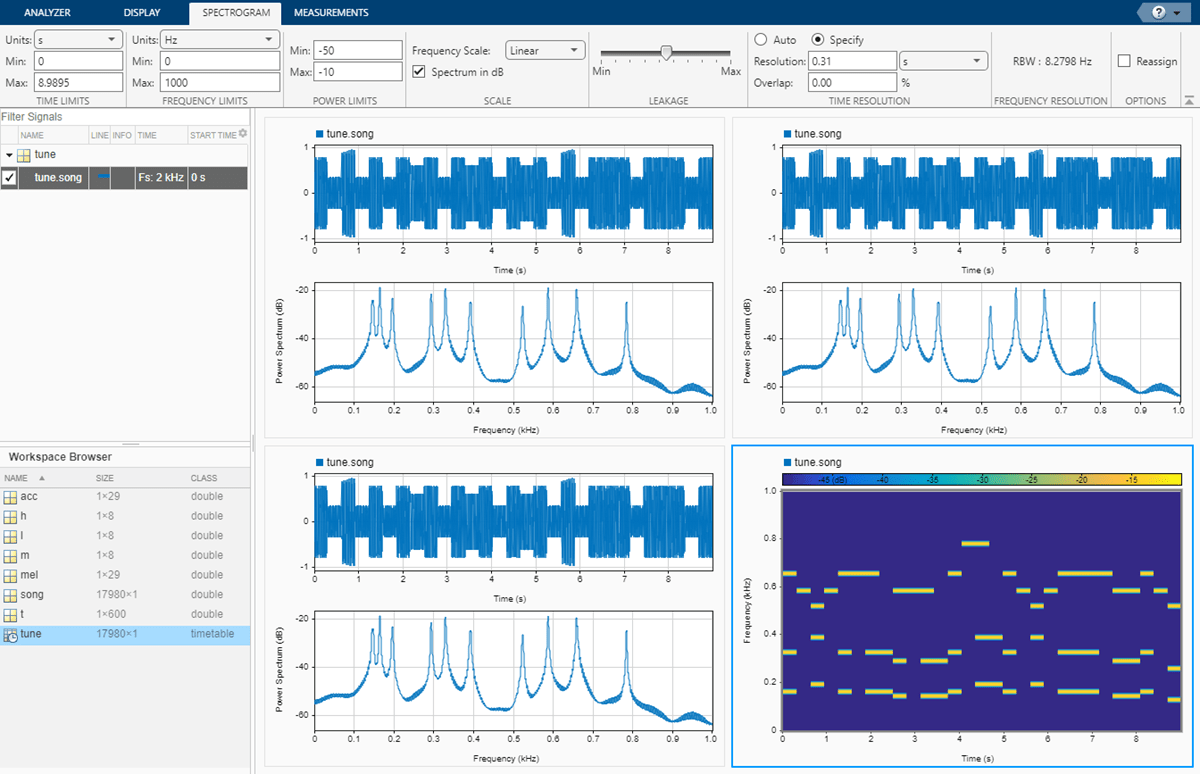On the Analyzer tab, click Duplicate three times to create three copies of the song. Rename the copies as high, medium, and low by double-clicking the Name column in the Signal table. Move the copies to the top two and lower left displays.

Preprocess the duplicate signals using filters.

1. Select the high signal by clicking its name in the Signal table. On the Analyzer tab, click Highpass. On the Highpass tab that appears, enter a passband frequency of 450 Hz and increase the steepness to 0.95. Click Highpass.

2. Select the medium signal by clicking its name in the Signal table. On the Analyzer tab, click Preprocessing ▼ and select Bandpass. On the Bandpass tab that appears, enter 230 Hz and 450 Hz as the lower and upper passband frequencies, respectively. Increase the steepness to 0.95. Click Bandpass.

3. Select the low signal by clicking its name in the Signal table. On the Analyzer tab, click Lowpass. On the Lowpass tab that appears, enter a passband frequency of 230 Hz and increase the steepness to 0.95. Click Lowpass.On each of the three displays containing filtered signals:

1. Remove the original signal by clearing the check box next to its name.

2. On the Display tab, click Time-Frequency to add a spectrogram view and click Time to remove the time view.

3. On the Spectrogram tab, specify a time resolution of 0.31 second and 0% overlap between adjoining segments. Set the Power Limits to $-50$ dB and $-10$ dB.Select the three filtered signals by clicking their Name column in the Signal table. On the Analyzer tab, click Export and save the signals to a MAT-file called music.mat. In MATLAB, load the file to the workspace. Plot the spectra of the three signals.

pspectrum(low)
hold on
pspectrum(medium)
pspectrum(high)
hold off% To hear the different voices, type
% sound(low.low,fs), pause(5), sound(medium.medium,fs), pause(5), sound(high.high,fs)

This example simulates the different steps of a basic communication process. Communication systems work by modulating chunks of information into a higher carrier frequency, transmitting the modulated signals through a noisy physical channel, receiving the noisy waveforms, and demodulating the received signals to reconstruct the initial information.

All the information carried in a real-valued signal $\mathit{s}\left(\mathit{t}\right)$ can be represented by a corresponding lowpass complex envelope:

$\mathit{s}\left(\mathit{t}\right)=\mathrm{Re}\left\{\mathit{g}\left(\mathit{t}\right){\mathit{e}}^{\mathit{j}2\pi {\mathit{f}}_{\mathrm{c}}\mathit{t}}\right\}=\mathit{i}\left(\mathit{t}\right)\mathrm{cos2}\text{\hspace{0.17em}}\pi \text{\hspace{0.17em}}{\mathit{f}}_{\mathit{c}}\mathit{t}+\mathit{q}\left(\mathit{t}\right)\mathrm{sin2}\text{\hspace{0.17em}}\pi \text{\hspace{0.17em}}{\mathit{f}}_{\mathit{c}}\mathit{t}$.

In this equation:

• ${\mathit{f}}_{\mathrm{c}}$ is the carrier frequency.

• $Re$ represents the real part of a complex-valued quantity.

• $\mathit{g}\left(\mathit{t}\right)=\mathit{i}\left(\mathit{t}\right)+\mathit{jq}\left(\mathit{t}\right)$is the complex envelope of $\mathit{s}\left(\mathit{t}\right)$.

• $\mathit{i}\left(\mathit{t}\right)$ is the inphase component of the complex envelope.

• $\mathit{q}\left(\mathit{t}\right)$ is the quadrature component of the complex envelope.

The complex envelope is modulated to the carrier frequency and sent through the channel. At the receiver, the noisy waveform is demodulated using the carrier frequency.

The phase variation due to the carrier frequency is predictable and thus does not convey any information. The complex envelope does not include the phase variation and can be sampled at a lower rate.

Generate a signal whose complex envelope consists of a sinusoid and a chirp. The inphase component is a sinusoid with a frequency of 19 Hz. The quadrature component is a quadratic chirp whose frequency ranges from 61 Hz to 603 Hz. The signal is sampled at 2 kHz for 1 second.

fs = 2e3;
t = (0:1/fs:1-1/fs)';
inph = sin(2*pi*19*t);

Compute the complex envelope and store it as a MATLAB® timetable of sample rate fs.

env = inph + 1j*quad;
g = timetable(env,'SampleRate',fs);

Open Signal Analyzer and drag the complex envelope from the Workspace browser to the Signal table. The display shows the inphase and quadrature components of the envelope as lines of the same hue and saturation, but different luminosity. The first line color represents the inphase component and the second line color represents the quadrature component. Click Spectrum ▼ on the Display tab and select Spectrum. The app displays a set of axes with the signal spectrum. The complex envelope has a two-sided spectrum, displayed as a line of the same color of the inphase component of the complex envelope.On the Display tab, click Panner to activate the panner. Use the panner to create a zoom window between 300 ms and 720 ms. Drag the zoom window so that it is centered at 0 Hz. The spectrum has an impulse at 0.19 kHz and a wider tapering profile at higher frequencies. The negative-frequency region of the spectrum is a mirror image of the positive-frequency region.Modulate the signal using a carrier frequency of 200 Hz. Multiply by $\sqrt{2}$ so that the power of the modulated signal equals the power of the original signal. Add white Gaussian noise such that the signal-to-noise ratio is 40 dB.

fc = 200;
mod = sqrt(2)*real(env.*exp(2j*pi*fc*t));

SNR = 40;
mod = mod + randn(size(mod))*std(mod)/db2mag(SNR);
s = timetable(mod,'SampleRate',fs);

Click Display Grid ▼ to add a second display. Drag the modulated signal to the Signal table and enter the time information. The modulation has moved the spectrum to positive frequencies centered on the carrier frequency.Calculate the analytic signal and demodulate the signal by multiplying the analytic signal with a complex-valued negative exponential of frequency 200 Hz.dem = hilbert(mod).*exp(-2j*pi*fc*t)/sqrt(2);

Click Display Grid ▼ to create a three-by-one grid of displays. Drag the demodulated signal to the Signal table. Add time information to the complex envelope by clicking Time Values in the Analyzer tab. The two-sided spectrum shows the recovered inphase and quadrature components of the baseband signal.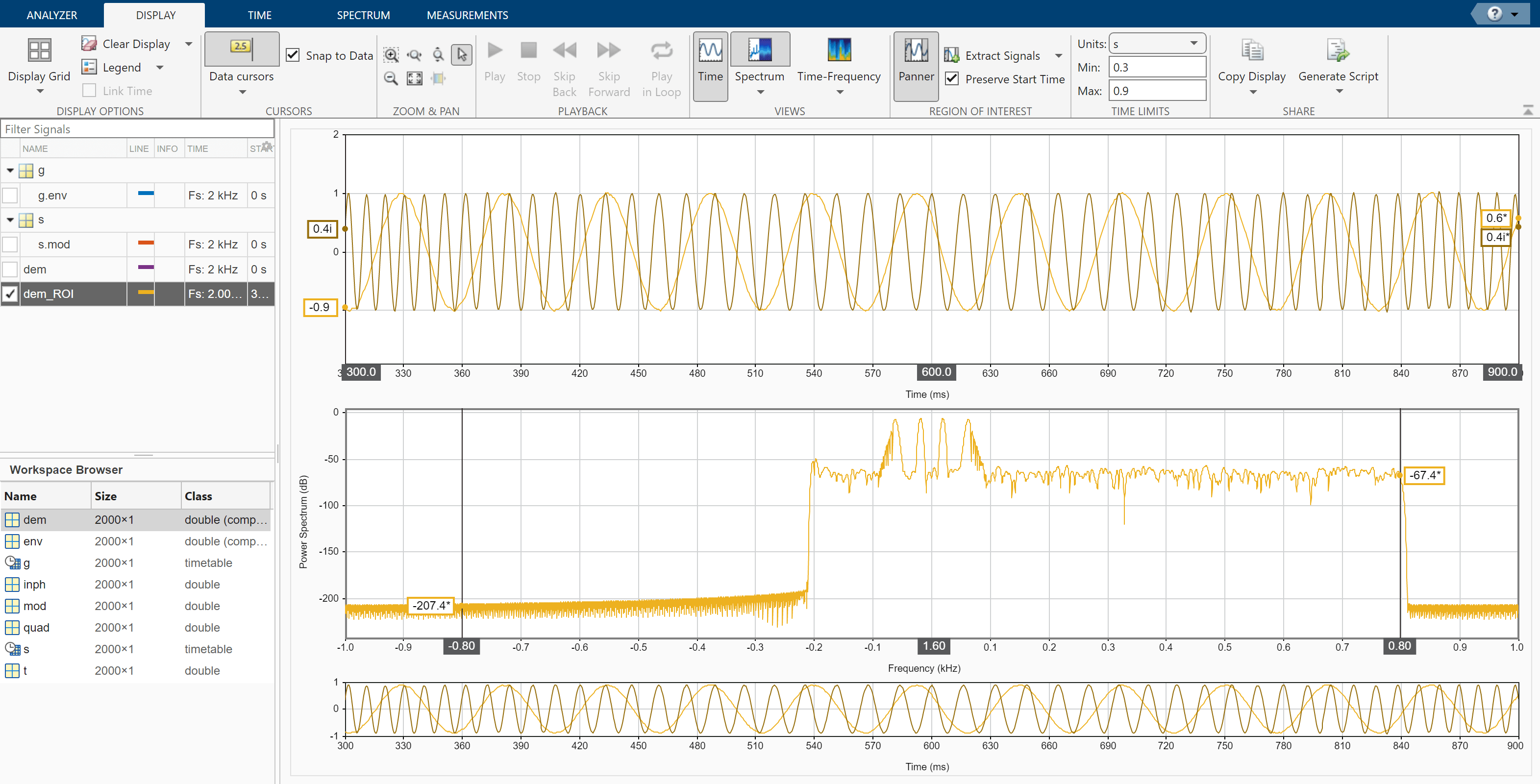Click Display Grid ▼ to create a one-by-one grid of displays and plot the demodulated signal. Click Data Cursors ▼ and select Two. Place the time-domain cursors at 300 ms and 900 ms, so they enclose the spectral peaks. Click Extract Signals ▼ and select Between Time Cursors. Check the Preserve Start Time box. Clear the display and plot the extracted signal. The app extracts both inphase and quadrature components of the demodulated signal in the region of interest. Select the extracted signal by clicking its Name column in the Signal table. On the Analyzer tab, click Export and save the signal to a MAT-file called dem_ROI.mat.Load the dem_ROI file to the workspace. Compute the demodulated inphase and quadrature components by taking the real and imaginary parts of the extracted signal. Store the time information of the extracted signal in a time variable t_dem.

inph_dem = real(dem_ROI);
t_dem = 0.3+(0:length(dem_ROI)-1)/fs;

Compare the transmitted waveforms and the extracted regions of interest. Also compare their spectra.

subplot(2,1,1)
plot(t,inph,t_dem,inph_dem,'--')
legend('Transmitted Inphase Signal','Received Inphase Signal')

subplot(2,1,2)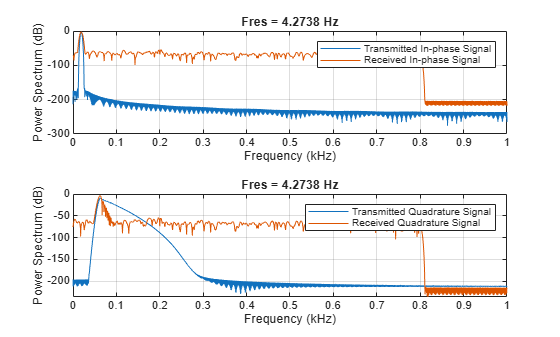figure
subplot(2,1,1)
pspectrum(inph,fs)
hold on
pspectrum(inph_dem,fs)
legend('Transmitted Inphase Signal','Received Inphase Signal')
hold off

subplot(2,1,2)
hold on
hold offLoad a datafile containing an echolocation pulse emitted by a big brown bat (Eptesicus fuscus) and measured with a sampling interval of 7 microseconds. Create a MATLAB® timetable using the signal and the time information.

t = (0:length(batsignal)-1)*DT;
sg = timetable(seconds(t)',batsignal);

Open Signal Analyzer and drag the timetable from the Workspace browser to the Signal table. Click Display Grid ▼ to create two side-by-side displays. Select each display and click the Time-Frequency button to add a spectrogram view.

Drag the timetable to both displays.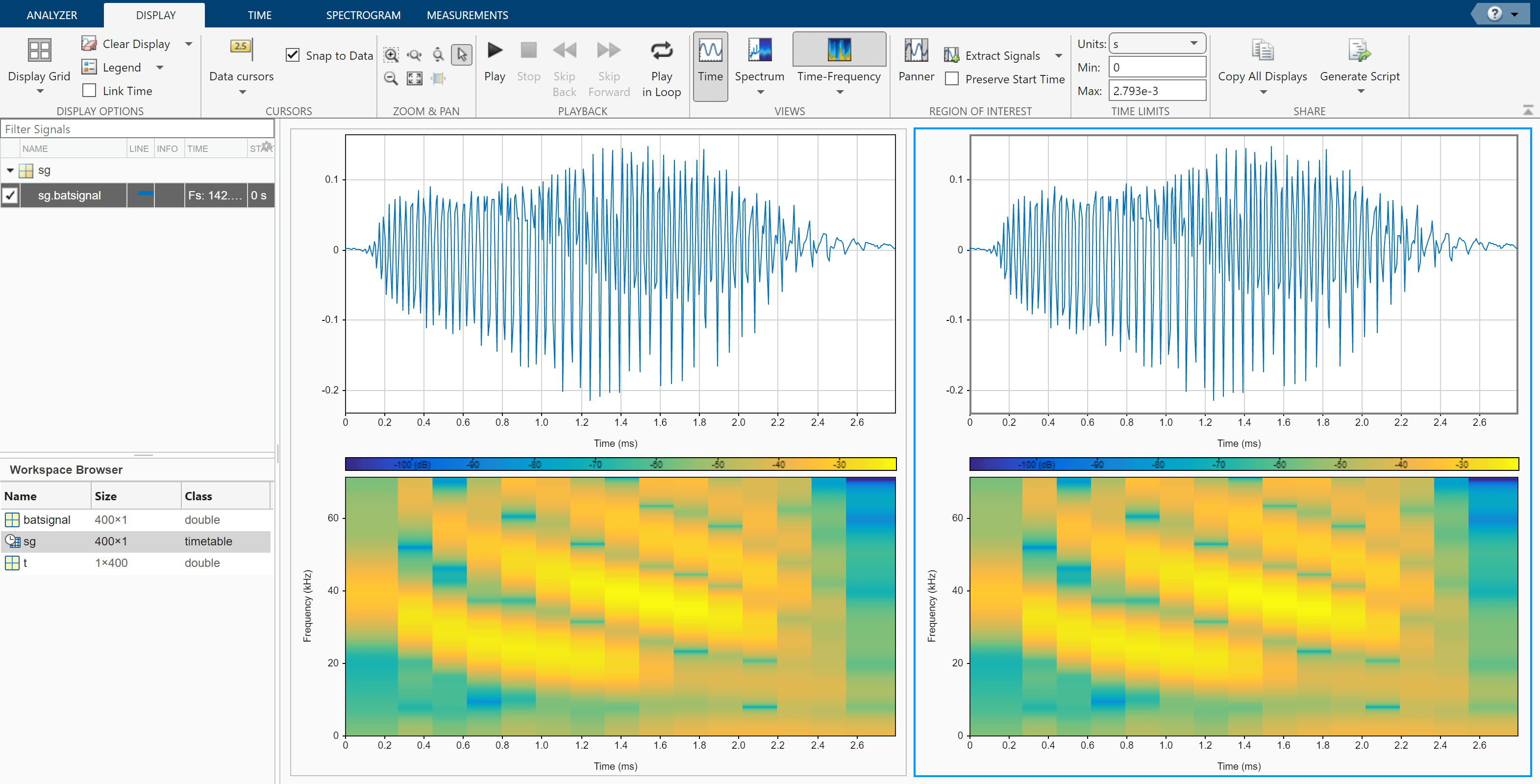Select the Spectrogram tab. On the display at right, check Reassign. For each display:

• Set the time resolution to 280 microseconds and specify 85% overlap between adjoining segments.

• Use the Leakage slider to increase the leakage until the RBW is about 4.5 kHz.

• Set the power limits to –45 dB and –20 dB.The reassigned spectrogram clearly shows three time-frequency ridges. To track the ridges, select the display at right. On the Display tab, click Generate Script and select Spectrogram Script. The script appears in the Editor.

% Compute spectrogram

% Generated by MATLAB(R) 9.7 and Signal Processing Toolbox 8.2.
% Generated on: 26-Dec-2018 17:21:44

% Parameters
timeLimits = seconds([0 0.002793]); % seconds
frequencyLimits = [0 71428.57]; % Hz
leakage = 0.9;
timeResolution = 0.00028; % seconds
overlapPercent = 85;
reassignFlag = true;

%%
% Index into signal time region of interest
sg_batsignal_ROI = sg(:,'batsignal');
sg_batsignal_ROI = sg_batsignal_ROI(timerange(timeLimits(1),timeLimits(2),'closed'),1);

% Compute spectral estimate
% Run the function call below without output arguments to plot the results
[P,F,T] = pspectrum(sg_batsignal_ROI, ...
'spectrogram', ...
'FrequencyLimits',frequencyLimits, ...
'Leakage',leakage, ...
'TimeResolution',timeResolution, ...
'OverlapPercent',overlapPercent, ...
'Reassign',reassignFlag);

Run the script. Plot the reassigned spectrogram.

mesh(seconds(T),F,P)
xlabel('Time')
ylabel('Frequency')
axis tight
view(2)
colormap pink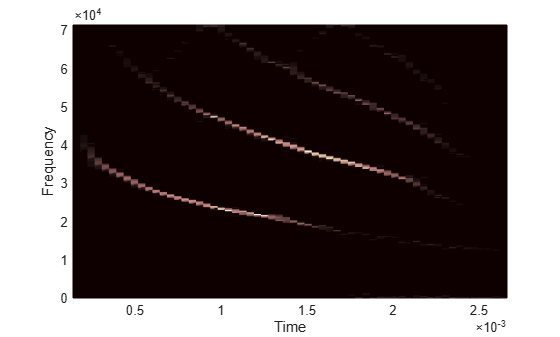Use the tfridge function to track the ridges.

[fridge,~,lridge] = tfridge(P,F,0.01,'NumRidges',3,'NumFrequencyBins',10);

hold on
plot3(seconds(T),fridge,P(lridge),':','linewidth',3)
hold off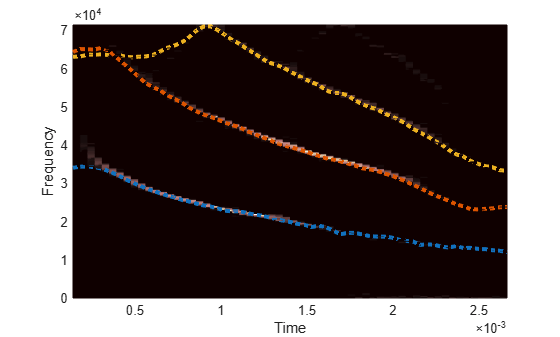Thanks to Curtis Condon, Ken White, and Al Feng of the Beckman Center at the University of Illinois for the bat data and permission to use it in this example.

Sensors can return clipped readings if the data are larger than a given saturation point. To reconstruct the readings, you can fit a polynomial through the points adjacent to the saturated intervals. Write a function that performs the reconstruction and integrate it into Signal Analyzer.

Generate a three-channel signal sampled at 1 kHz for 14 seconds. The signal has several peaks of varying sizes and shapes. A sensor that reads the signal saturates at 0.1 V.

fs = 1000;
t = 0:1/fs:14-1/fs;

0.85*besselj(0,5*(sin(2*pi*(t+1.5).^2/20).^2)).*sin(2*pi*t/9)]';

sigsat = sig;
stv = 0.1;
sigsat(sigsat >= stv) = stv;

Open Signal Analyzer and drag the original signal and the saturated signal to the Signal table. Drag each original and saturated channel to its own display.Write a function that uses a polynomial to reconstruct the signal peaks:

• The first input argument, x, is the input signal. This argument must be a vector and is treated as a single channel.

• The second input argument, tIn, is a vector of time values. The vector must have the same length as the signal. If the input signal has no time information, the function reads this argument as an empty array.

• Use varargin to specify additional input arguments. If you do not have additional input arguments, you can omit varargin. Enter the additional arguments as an ordered comma-separated list in the Preprocess tab.

• The first output argument, y, is the preprocessed signal.

• The second output argument, tOut, is a vector of output time values. If the input signal has no time information, tOut is returned as an empty array.

• To implement your algorithm, you can use any MATLAB® or Signal Processing Toolbox™ function.

function [y,tOut] = declip(x,tIn,varargin)
% Declip saturated signal by fitting a polynomial

% Initialize the output signal

y = x;

% For signals with no time information, use sample numbers as abscissas

if isempty(tIn)
tOut = [];
t = (1:length(x))';
else
t = tIn;
tOut = t;
end

% Specify the degree of the polynomial as an optional input argument
% and provide a default value of 4

if nargin<3
ndx = 4;
else
ndx = varargin{1};
end

% To implement your algorithm, you can use any MATLAB or Signal
% Processing Toolbox function

% Find the intervals where the signal is saturated and generate an
% array containing the interval endpoints
idx = find(x==max(x));
fir = [true;diff(idx)~=1];
ide = [idx(fir) idx(fir([2:end 1]))];
% For each interval, fit a polynomial of degree ndx over the ndx+1 points
% before the interval and the ndx+1 points after the interval
for k = 1:size(ide,1)
bef = ide(k,1); aft = ide(k,2);
intv = [bef-1+(-ndx:0) aft+1+(0:ndx)];
[pp,~,mu] = polyfit(t(intv),x(intv),ndx);
y(bef:aft) = polyval(pp,t(bef:aft),[],mu);
end

end

Add the function to Signal Analyzer as a custom preprocessing function. On the Analyzer tab, click Preprocessing ▼ and select Add Custom Function. Input the function name and description. Paste the text of your function in the editor window that appears. Save the file. The function appears in the preprocessing gallery.Demonstrate that the function you created reconstructs the saturated regions.

1. Select the first channel of the saturated signal in the Signal table.

2. On the Analyzer tab, click Preprocessing ▼ and select declip.

3. On the Preprocessing tab that appears, click Preprocess.

Verify that the preprocessing function works when the signals have time information.

1. Select sig and sigsat in the Signal table. Do not select individual channels.

2. On the Analyzer tab, click Time Values, select Sample Rate and Start Time, and specify fs as the sample rate.

3. The signal in the top panel, including the reconstructed regions, has time information.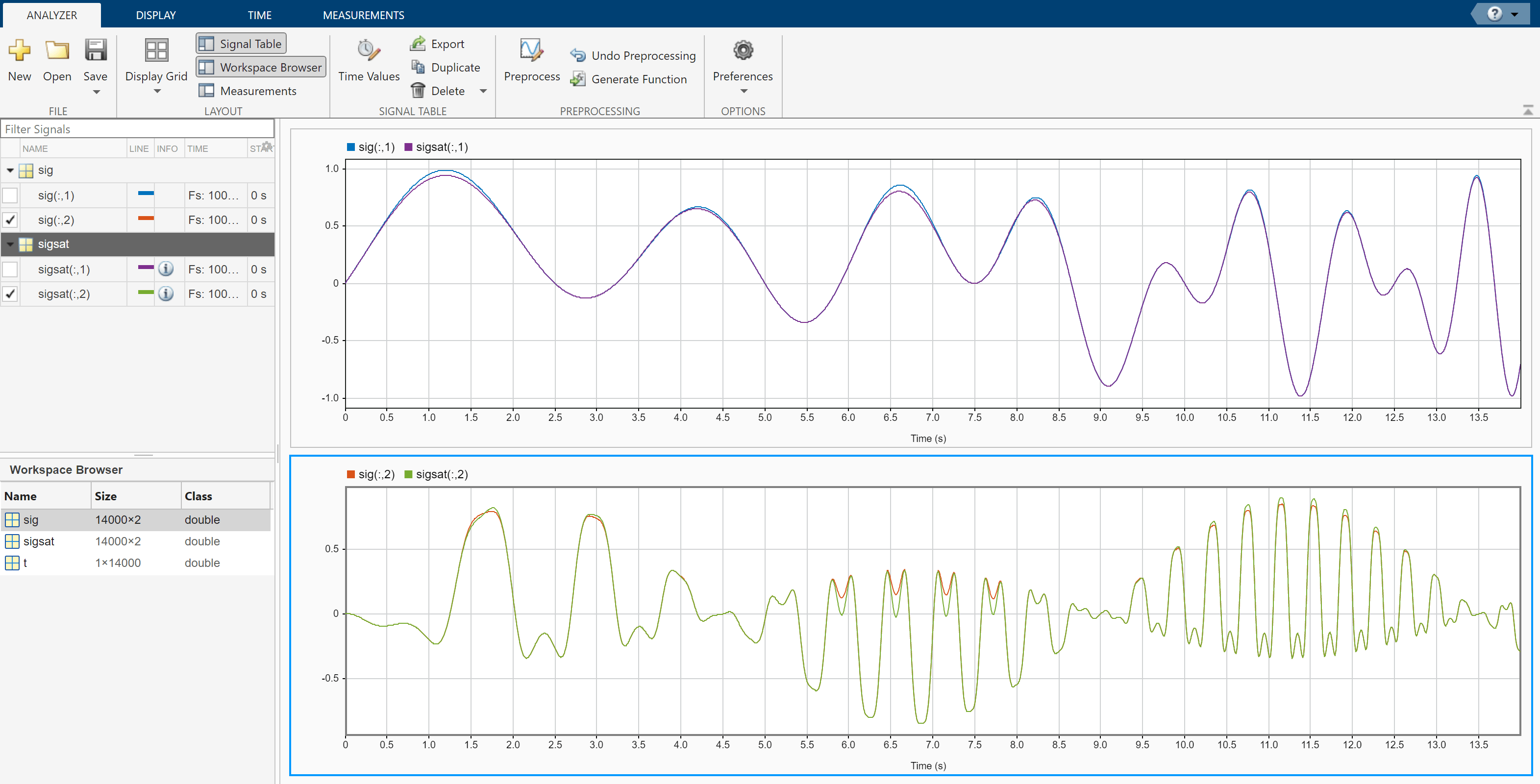Check that the function works when you specify optional inputs.

1. Select the second and third channels of the saturated signal in the Signal table.

2. On the Preprocessing tab, enter 8 in the Arguments field and click Preprocess. The preprocessing function uses a polynomial of degree 8 to reconstruct the saturated regions.Use Signal Analyzer to compute the envelope spectrum of a bearing vibration signal and look for defects. Generate MATLAB® scripts and functions to automate the analysis.

Generate Bearing Vibration Data

A bearing with the dimensions shown in the figure is driven at ${\mathit{f}}_{0}=25$ cycles per second. An accelerometer samples the bearing vibrations at 10 kHz.Generate vibration signals from two defective bearings using the bearingdata function at the end of the example. In one of the signals, xBPFO, the bearing has a defect in the outer race. In the other signal, xBPFI, the bearing has a defect in the inner race. For more details on modeling and diagnosing defects in bearings, see Vibration Analysis of Rotating Machinery and envspectrum.

[t,xBPFO,xBPFI,bpfi] = bearingdata;

Compute Envelope Spectrum Using Signal Analyzer

Open Signal Analyzer and drag the BPFO signal to a display. Add time information to the signal by selecting it in the Signal table and clicking the Time Values button on the Analyzer tab. Select the Sample Rate and Start Time option and enter the 10 kHz sample rate.

On the Display tab, click Spectrum to open a spectrum view. The spectrum of the vibration signal shows BPFO harmonics modulated by the 3 kHz impact frequency. At the low end of the spectrum, the driving frequency and its orders obscure other features.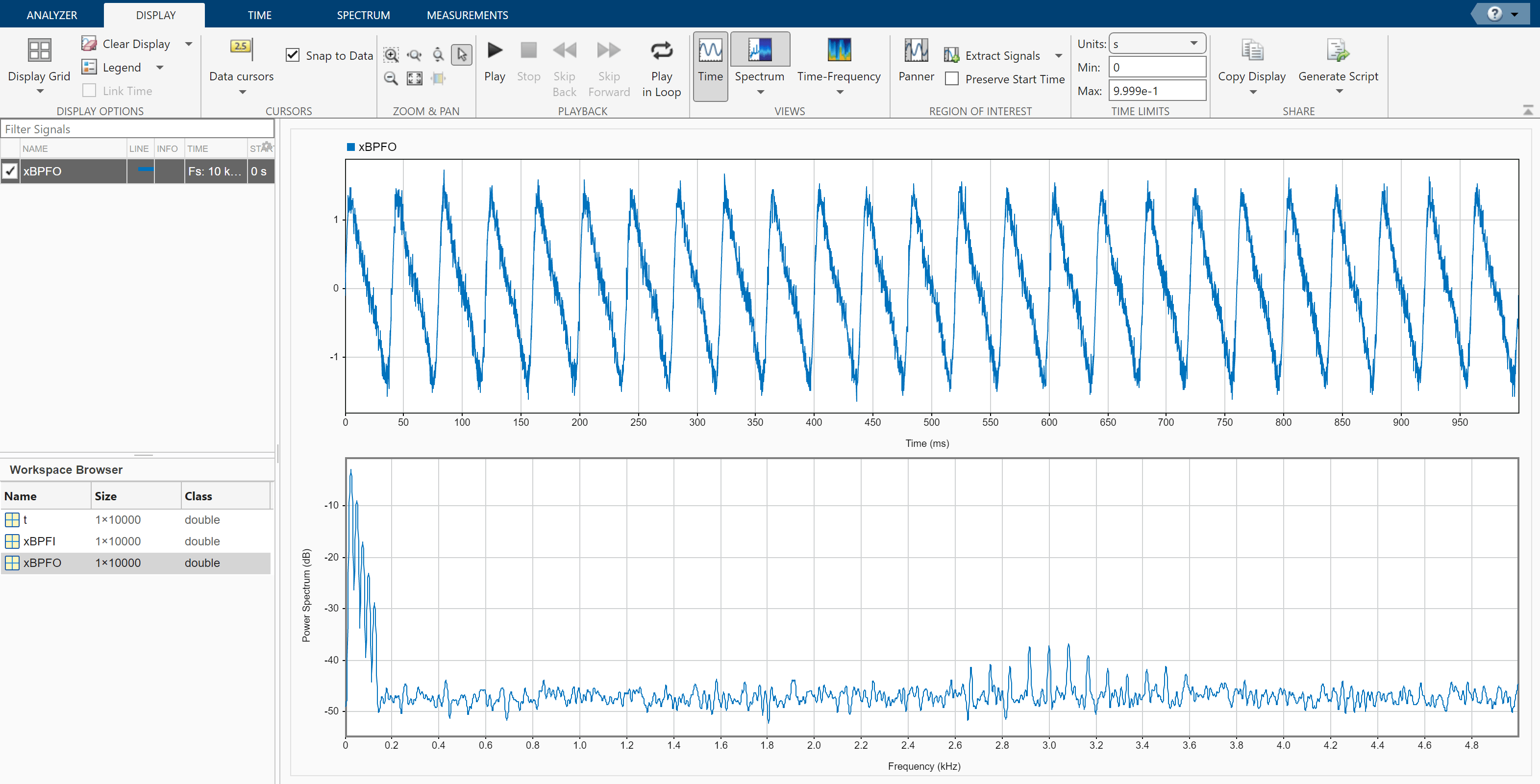Select the signal and, on the Analyzer tab, click Duplicate to generate a copy of it. Give the new signal the name envspec and drag it to the display. Compute the envelope spectrum of the signal using the Hilbert transform:

1. Remove the DC value of the signal. On the Analyzer tab, click Preprocessing ▼ and select Detrend. On the Detrend tab that appears, select Constant as the method. Click Detrend.

2. Bandpass-filter the detrended signal. On the Analyzer tab, click Preprocessing ▼ and select Bandpass. On the Bandpass tab that appears, enter 2250 Hz and 3750 Hz as the lower and upper passband frequencies, respectively. Click Bandpass.

3. Compute the envelope of the filtered signal. On the Analyzer tab, click Preprocessing ▼ and select Envelope. On the Envelope tab that appears, select Hilbert as the method. Click Envelope.

4. Remove the DC value of the envelope using Detrend.

The envelope spectrum appears in the spectrum view of the display. The envelope spectrum clearly displays the BPFO harmonics.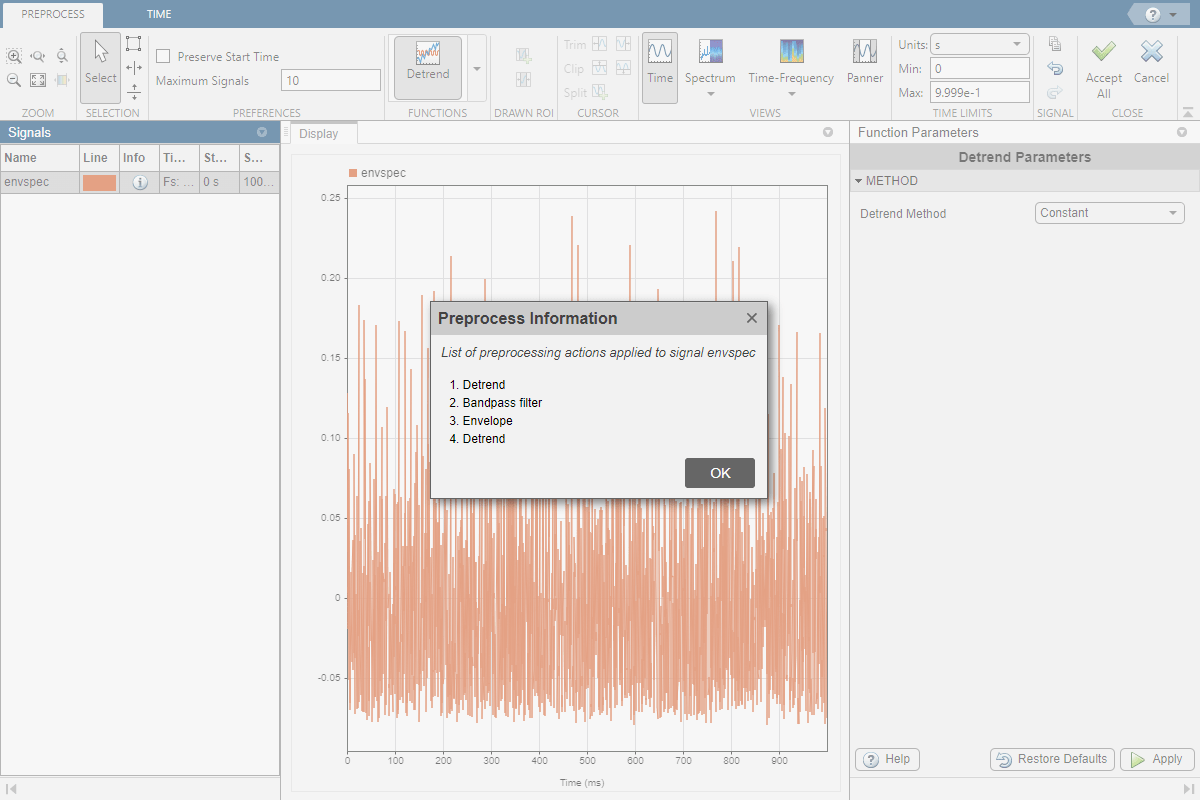Steps to Create an Integrated Analysis Script

The computation of the envelope spectrum can get tedious if it has to be repeated for many different bearings. Signal Analyzer can generate MATLAB® scripts and functions to help you automate the computation.

As an exercise, repeat the previous analysis for the BPFI signal. Signal Analyzer generates two components useful for the automation:

1. A function that preprocesses the signal by detrending it, filtering it, and computing its envelope

2. A script that computes the envelope spectrum

To create the integrated analysis script, put the preprocessing function and the plotting script together unchanged in a single file. (Alternatively, you can save functions in separate files.)

• If you save the script and the function in a single MATLAB® script, keep in mind that functions must appear at the end.

• You must add the keyword end at the end of each function.

1. Create Preprocessing Function

Initially, create the function that reproduces the preprocessing steps. Select the envspec signal. On the Analyzer tab, click Generate Function. The function, called preprocess by default, appears in the Editor. Save the generated function at the end of your integrated analysis script. The function expects a second argument specifying the time information. Preprocess the BPFI signal using the function.

envspec = preprocess(xBPFI,t);

2. Create Spectrum Script

In the app, remove the unprocessed signal from the display by clearing the check box next to its name. On the Display tab, click Generate Script ▼ and select Spectrum Script. The script appears in the Editor. Include the generated code in your integrated analysis script. When you run the analysis script, the generated spectrum script computes the envelope spectrum of the preprocessed BPFI signal.

% Compute power spectrum

% Generated by MATLAB(R) 9.6 and Signal Processing Toolbox 8.2.
% Generated on: 12-Nov-2018 15:13:34

% Parameters
timeLimits = [0 0.9999]; % seconds
frequencyLimits = [0 5000]; % Hz

%%
% Index into signal time region of interest
envspec_ROI = envspec(:);
sampleRate = 10000; % Hz
startTime = 0; % seconds
minIdx = ceil(max((timeLimits(1)-startTime)*sampleRate,0))+1;
maxIdx = floor(min((timeLimits(2)-startTime)*sampleRate,length(envspec_ROI)-1))+1;
envspec_ROI = envspec_ROI(minIdx:maxIdx);

% Compute spectral estimate
% Run the function call below without output arguments to plot the results
[Penvspec_ROI, Fenvspec_ROI] = pspectrum(envspec_ROI,sampleRate, ...
'FrequencyLimits',frequencyLimits);

3. Plot Envelope Spectrum

Plot the envelope spectrum. Compare the peak locations to the frequencies of the first ten BPFI harmonics. You can also plot the envelope spectrum using the pspectrum command with no output arguments.

plot(Fenvspec_ROI,(Penvspec_ROI))
hold on
[X,Y] = meshgrid((1:10)*bpfi,ylim);
plot(X,Y,':k')
hold off
xlim([0 10*bpfi])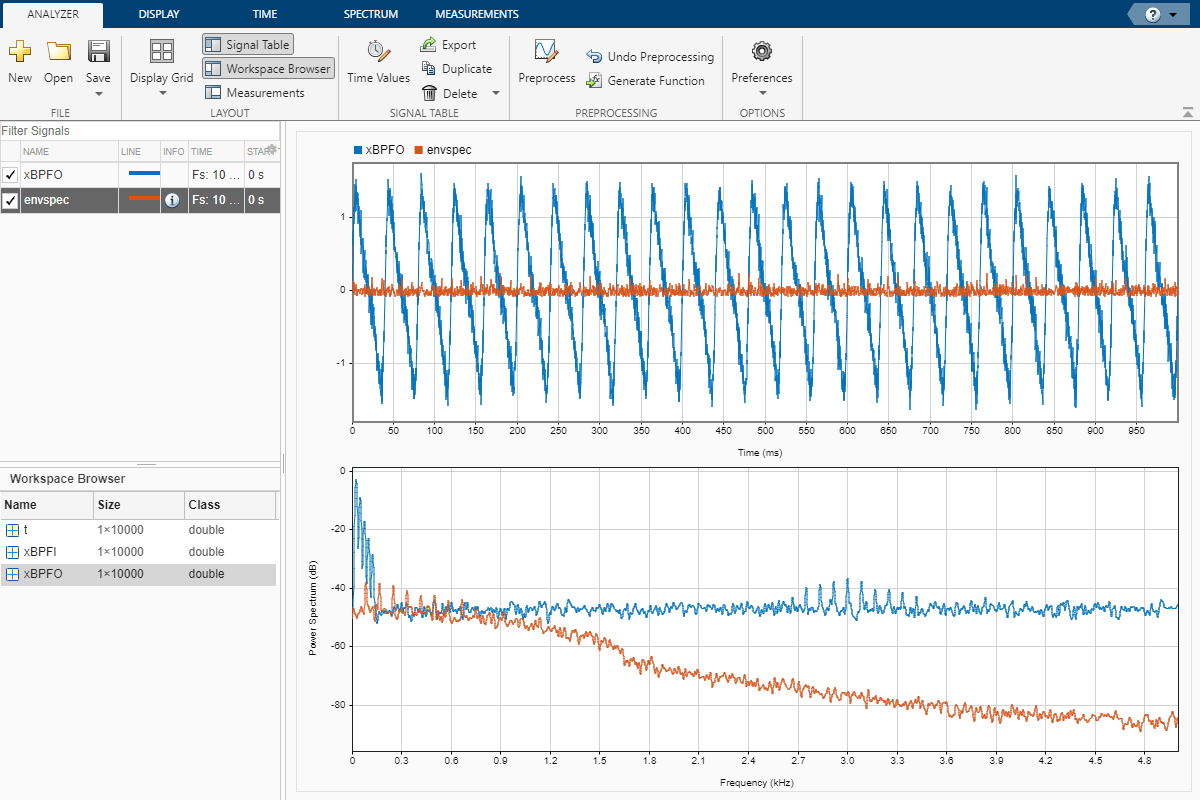Function Code

Signal Preprocessing Function

The signal preprocessing function generated by the app combines detrending, bandpass filtering, and envelope computation.

function y = preprocess(x,tx)
%  Preprocess input x
%    This function expects an input vector x and a vector of time values
%    tx. tx is a numeric vector in units of seconds.

% Generated by MATLAB(R) 9.6 and Signal Processing Toolbox 8.2.
% Generated on: 12-Nov-2018 15:09:44

y = detrend(x,'constant');
Fs = 1/mean(diff(tx)); % Average sample rate
y = bandpass(y,[2250 3750],Fs,'Steepness',0.85,'StopbandAttenuation',60);
[y,~] = envelope(y);
y = detrend(y,'constant');
end

Bearing Data Generating Function

The bearing has pitch diameter $\mathit{p}=12$ cm and a bearing contact angle $\theta =0$. Each of the $\mathit{n}=8$ rolling elements has a diameter $\mathit{d}=2$ cm. The outer race remains stationary as the inner race is driven at ${\mathit{f}}_{0}=25$ cycles per second. An accelerometer samples the bearing vibrations at 10 kHz.

function [t,xBPFO,xBPFI,bpfi] = bearingdata

p = 0.12;
d = 0.02;
n = 8;
th = 0;
f0 = 25;
fs = 10000;

For a healthy bearing, the vibration signal is a superposition of several orders of the driving frequency, embedded in white Gaussian noise.

t = 0:1/fs:1-1/fs;
z = [1 0.5 0.2 0.1 0.05]*sin(2*pi*f0*[1 2 3 4 5]'.*t);

xHealthy = z + randn(size(z))/10;

A defect in the outer race causes a series of 5 millisecond impacts that over time result in bearing wear. The impacts occur at the ball pass frequency outer race (BPFO) of the bearing,

$\mathrm{BPFO}=\frac{1}{2}{\mathit{nf}}_{0}\left[1-\frac{\mathit{d}}{\mathit{p}}\mathrm{cos}\text{\hspace{0.17em}}\theta \right]$.

Model the impacts as a periodic train of 3 kHz exponentially damped sinusoids. Add the impacts to the healthy signal to generate the BPFO vibration signal.

bpfo = n*f0/2*(1-d/p*cos(th));

tmp = 0:1/fs:5e-3-1/fs;
xmp = sin(2*pi*3000*tmp).*exp(-1000*tmp);

xBPFO = xHealthy + pulstran(t,0:1/bpfo:1,xmp,fs)/4;

If the defect is instead in the inner race, the impacts occur at a frequency

$\mathrm{BPFI}=\frac{1}{2}{\mathit{nf}}_{0}\left[1+\frac{\mathit{d}}{\mathit{p}}\mathrm{cos}\text{\hspace{0.17em}}\theta \right]$.

Generate the BPFI vibration signal by adding the impacts to the healthy signals.

bpfi = n*f0/2*(1+d/p*cos(th));

xBPFI = xHealthy + pulstran(t,0:1/bpfi:1,xmp,fs)/4;

end

Programmatic Use

expand all

signalAnalyzer opens the Signal Analyzer app.

signalAnalyzer(sig) opens the Signal Analyzer app and imports and plots the signal sig. If the app is already open, then it plots sig in the current display. If sig is already plotted but has changed, then the function call updates the plot.

sig can be a variable in the workspace or a MATLAB expression. sig can be:

• A vector or a matrix with independent signals in each column.

• A timetable with time values specified as durations.

• A timeseries object.

See Data Types Supported by Signal Analyzer for more details.

By default, the app plots the signal as a function of sample index. If you provide time information, or if the signal has inherent time information, then the app plots the signal as a function of time.

signalAnalyzer(sig1,...,sigN) imports N signal vectors or matrices and plots them in the current display. The app does not support importing signals with inherent time information and signals without inherent time information in the same function call.

signalAnalyzer(___,'SampleRate',fs) specifies a sample rate, fs, as a positive scalar expressed in Hz. The app uses the sample rate to plot one or more signals against time, assuming a start time of zero. You can specify a sample rate for signals with no inherent time information.

signalAnalyzer(___,'SampleTime',ts) specifies a sample time, ts, as a positive scalar expressed in seconds. The app uses the sample time to plot one or more signals against time, assuming a start time of zero. You can specify a sample time for signals with no inherent time information.

signalAnalyzer(___,'StartTime',st) specifies a signal start time, st, as a scalar expressed in seconds. If you do not specify a sample rate or sample time, then the app assumes a sample rate of 1 Hz. You can specify a start time for signals with no inherent time information.

signalAnalyzer(___,'TimeValues',tv) specifies a vector, tv, with time values corresponding to the data points. tv can be a real numeric vector with values expressed in seconds. tv can also be a duration array. The values in tv must be unique and cannot be NaN, but they need not be uniformly spaced. All input signals must have the same length as tv. You can specify a vector of time values for signals with no inherent time information.

Filtering and scalogram view do not support nonuniformly sampled signals.